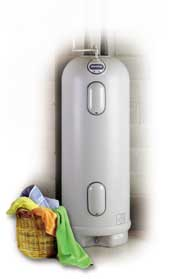A# water heating costs, estimates, and comparisons

When considering a water heater model for your home, estimate its annual operating cost. Then, compare costs with other more and/or less energy-efficient models. This will help you determine the energy savings and payback period of investing in a more energy-efficient model, which will probably have a higher purchase price.Before you can choose and compare the costs of various models, you need to determine the correct size water heater for your home.

## Calculating annual operating cost

To estimate the annual operating cost of a storage, demand (tankless or instaneous), or heat pump (not geothermal heat pump) water heater, you need to know the following about the model:

• energy factor (EF)
• fuel type and cost (your local utility can provide current rates)
•

Then, use the following calculations:

For gas and oil water heaters

You need to know the unit cost of fuel by BTU (British thermal unit) or therm. (1 therm = 100,000 BTU)

365 × 41045/EF × fuel cost (BTU) = estimated annual cost of operation

OR

365 × 0.4105/EF × fuel cost (therm) = estimated annual cost of operation

Where the constant of 41045 BTU/day (0.4105 therm/day) is the average daily energy use of a standard gas or oil water heater.

Example: A natural gas water heater with an EF of 0.57 and a fuel cost of \$0.00000619/Btu

365 × 41045/.57 × \$0.00000619 = \$163

For electric water heaters, including heat pump units

You need to know or convert the unit cost of electricity by kilowatt-hour (kWh).

365 × 12.03/EF × electricity cost by kWh = estimated annual cost of operation

Where the constant of 12.03 kWh/day is the average daily energy use of a standard electric hot water heater.

Example: A heat pump water heater with an EF of 2.0 and a electricity cost of \$0.0842/kWh

365 × 12.03/2.0 × \$0.0842 = \$185

## Comparing costs and determining payback

Once you know the purchase and annual operating costs of the water heater models you want to compare, you can use the table below to determine the cost savings and payback of the more energy-efficient model(s).

 Models Price of water heater EF Estimated annual operating cost Model A Model B (higher EF) Additional cost of more efficient model (Model B) Price of Model B - price of Model A = \$additional cost of Model B Estimated annual operating cost savings (Model B) Model B annual operating Cost - Model A annual operating cost = \$Model B's cost savings per year Payback period for Model B \$Additional cost of Model B/\$Model B's cost savings per year = payback period/years

Example

Comparison of two gas water heaters with a local fuel cost of .60 per therm.

 Models Price of water heater EF Estimated annual operating cost Model A \$165 .54 \$166 Model B \$210 .58 \$155 Additional cost of more efficient model (Model B) \$210-\$165=\$45 Estimated annual operating cost savings (Model B) \$166-\$155=\$11 per year Payback period for Model B \$45/\$11 per year = 4.1 years

## Other costs

If you want to include installation and maintenance costs, consult the manufacturer(s) and a qualified contractor to help estimate these costs. These costs will vary among system types and sometimes even from water heater model to model.